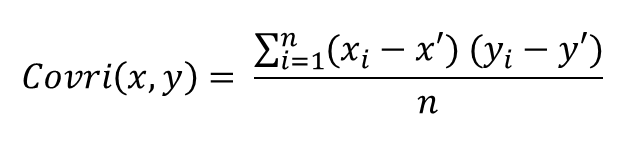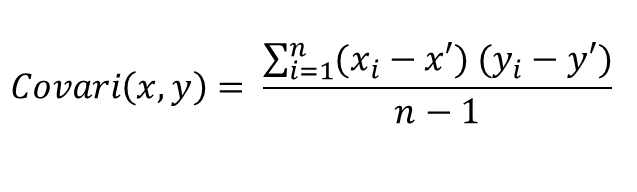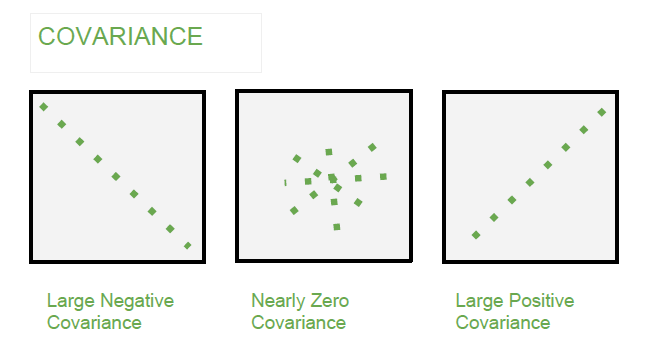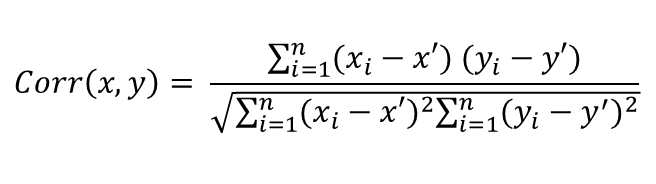GFG App
Open AppBrowser
Continue

# Mathematics | Covariance and Correlation

Covariance and Correlation are two mathematical concepts which are commonly used in the field of probability and statistics. Both concepts describe the relationship between two variables.

Covariance –

1. It is the relationship between a pair of random variables where change in one variable causes change in another variable.
2. It can take any value between -infinity to +infinity, where the negative value represents the negative relationship whereas a positive value represents the positive relationship.
3. It is used for the linear relationship between variables.
4. It gives the direction of relationship between variables.

Formula –
For Population:For SampleHere,
x’ and y’ = mean of given sample set
n = total no of sample
xi and yi = individual sample of set

Example –Correlation –

1. It show whether and how strongly pairs of variables are related to each other.
2. Correlation takes values between -1 to +1, wherein values close to +1 represents strong positive correlation and values close to -1 represents strong negative correlation.
3. In this variable are indirectly related to each other.
4. It gives the direction and strength of relationship between variables.

Formula –Here,
x’ and y’ = mean of given sample set
n = total no of sample
xi and yi = individual sample of set

Example –Covariance versus Correlation –

Covariance Correlation
Covariance is a measure of how much two random variables vary together Correlation is a statistical measure that indicates how strongly two variables are related.
involve the relationship between two variables or data sets involve the relationship between multiple variables as well
Lie between -infinity and +infinity Lie between -1 and +1
Measure of correlation Scaled version of covariance
provide direction of relationship provide direction and strength of relationship
dependent on scale of variable independent on scale of variable
have dimensions dimensionless
My Personal Notes arrow_drop_up